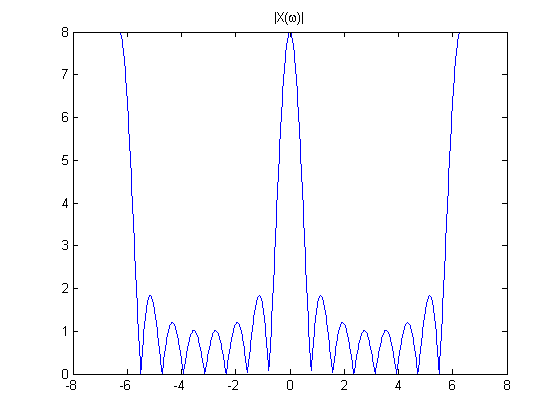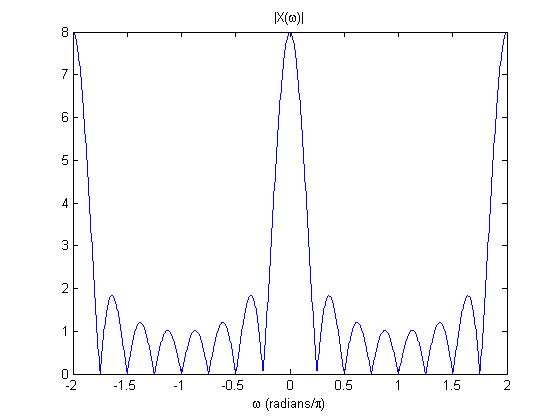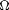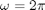# No units in the DTFT

OysterEngineer (I love that name!) asked some interesting questions in response to my last Fourier transform post, which concerned the relationship between the discrete-time Fourier transform (DTFT) and the discrete Fourier transform (DFT).

I'd like to first tackle OE's comments about units. Or, more specifically, the lack of units in my DTFT plots, such as this one:OE guesses that the units for my unlabeled horizontal axis above are radians/second.

The DTFT is unitless; there are no units of time or frequency. If I were going to label the horizontal axis, I would label it "radians." Because it is harder than it should be in MATLAB to label an axis in fractions of, I might do it like this:

M = 8;
w = linspace(-2*pi, 2*pi, 800);
X_dtft = (sin(w*M/2) ./ sin(w/2)) .* exp(-1j * w * (M-1) / 2);
plot(w/pi, abs(X_dtft))
title('|X(\omega)|')
xlabel('\omega (radians/\pi)')There is a scaling relationship between(radians) of the DTFT and the physical frequency(radians/second or Hz). I mentioned (cryptically) this scaling relationship in my post on the relationship between the DTFT and continuous-time Fourier transform, but I did not really explain it very well.

Here's how I recommend that you remember it:corresponds to the sampling frequency, andcorresponds to half the sampling frequency. If the sampling frequency is 4 kHz, thencorresponds to the physical frequency 2 kHz.

I learned digital signal processing (DSP) theory beginning in my senior year at Georgia Tech in 1986 and continuing through my graduate studies there. My impression is that a significant notational shift was occurring at around that time in DSP. You could see this shift in two books by Oppenheim and Schafer written at different times. In my first graduate DSP course in 1987 we used the book Digital Signal Processing, which was published in 1975. In the 1970s, DSP notation was all about sampled systems and Fourier transforms and Fourier series, virtually every equation was filled with T and Fs terms, and there was nothing called the discrete-time Fourier transform.

But later in my graduate studies I earned spare change by writing a couple of chapters of the solutions manual for the first edition of Discrete-Time Signal Processing by the same authors. In this book the discrete-time Fourier transform is fundamental and very few of the equations in the book have T and Fs terms. Notationally this is much more convenient. The mathematics of designing a half-band lowpass filter are identical whether your sampling frequency is 4 kHz or 200 GHz.

But it does mean that you have to remember whatmeans when you're looking at a DTFT plot.

I will try to be more careful in the future in labeling my plots.

Published with MATLAB® 7.9

|

### コメント

コメントを残すには、ここ をクリックして MathWorks アカウントにサインインするか新しい MathWorks アカウントを作成します。